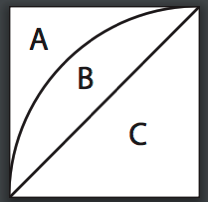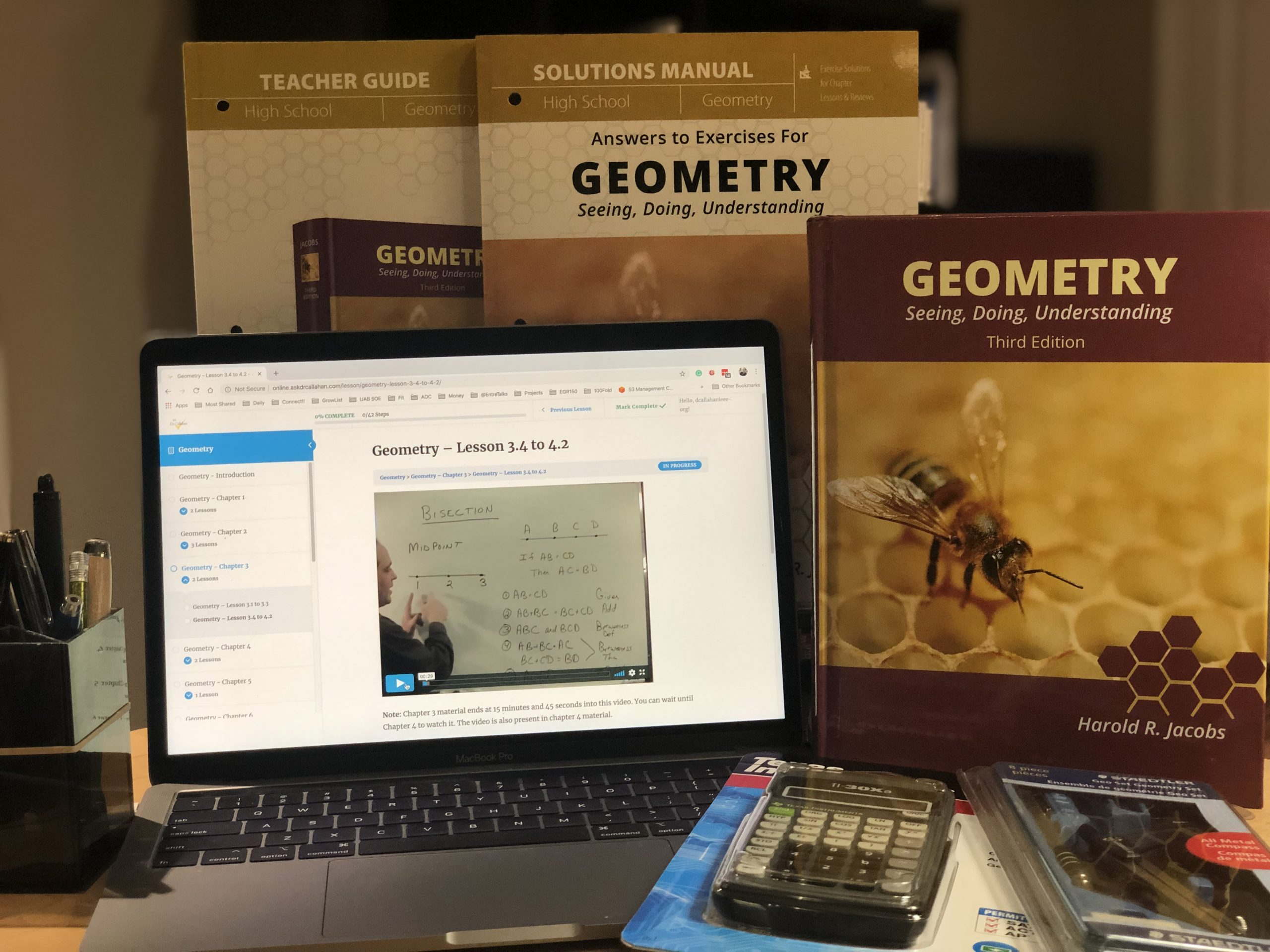Posted on

## Geometry Textbook Evaluations

Look for the following material or heading in the contents.

• Reasoning and Proof
· Proofs
· Deductive reasoning
· Direct and indirect proofs
• Lines
· Parallel and Perpendicular Lines
· Angles
• Triangles
· Congruent
· Isosceles
· Equilateral
· ASA and SAS
· Parallelograms
· Rectangles
· Squares
· Trapezoids
• Area
· Squares
· Rectangles
· Triangles
• Similarity
· Ratio and proportion
· Similar figures
• Right Triangle Trigonometry
· Pythagorean Theorem
· Proportions
· Tangent, Sine, and Cosine
• Surface Area and Volume
· Geometric solids
· Rectangular solids
· Spheres
• Circles
· Chords
· Tangents
• Transformations
· Reflections

Notes for use: One of the keys of geometry is learning deductive reasoning or how to do proofs. This can be a challenge to teach, so getting a teachers manual will really help here. The second main idea of geometry is getting used to thinking in space with shapes and the relationships between them. Lots of figures should be drawn.

Posted on

## Geometry Ch3 Algebra Review #16

The book has a problem

(x-3)(x^2 – 7x – 2)

which results in an answer of

x^3 – 10x^2 – 2x +19x + 6

The solution is incorrect in the new solutions manual ( the one with a bee on the cover)

However, it is correct in the old solutions manual -the one with a chess set on the cover.

Posted on

## Geometry Ch14 Test #4

Question: how do we work the problem on Chapter 14 test #4.

Since all 16 white regions are identical, we can find the area of one of them and multiply it by 16.

This figure represents one of the 16 small squares and one of the white regions.Since the diameter of the large circle in the figure shown on the test page is 4 units, the side of the square of one of the small squares is 1 unit.

We can find the area of region B by finding the area of regions B & C combined (a quarter of a circle) and subtracting the area of region C (a triangle):

> The area of B & C combined is1/4 (pi r squared) or 1/4 (pi 1 squared) = 1/4 pi

> The area of C is1/2 r squared or 1/2 1 squared = 1/2So B = (B + C) – C = 1/4 pi – 1/2 and the total area of the 16 white regions is16(1/4 pi – 1/2) = 4 pi – 8.

Posted on

## Geometry Ch10.4 Sett III #4

In Chp. 10, Lesson 4, Set III Question 4 the textbook asks “Why is QD/AC = DB/CD?”
Shouldn’t it be DB/CB instead of DB/CD?
Again you are correct. This is an error in the new printing of the 3rd edition by My Father’s World.
Posted on

## Geometry 10.2 Set III #4

Kerry wrote:
For Ch. 10, Lesson 2, Set III number 4 asks for rectangles with a ratio of 3/4.  The key says there are 4 rectangles that have dimensions of 1.5×2.  ABHG is correct, but the other three rectangles seem to be wrong.  The key lists BDJI (which doesn’t even make a rectangle), CEKI (which is a 1.5×3 rectangle), and DFLJ (which is also a 1.5×3 rectangle). Can you confirm the correct rectangles are ABHG, CDJI, and EFLK? I know it seems obvious, but just want to make sure I’m not missing something here.
Dr. Callahan Wrote:
You are correct. This looks like a typo. The original solutions manual (titled “Enhanced Teacher’s Guide for Geometry – ISBN: 978-0716756071) had it correct. I have noticed a few places that the answers in the newer solutions manual have typos.
Posted on

## Geometry Course Description for TranscriptIf you are looking for details on for a transcript for Jacobs Geometry Course, this description should do the trick.

Geometry is a branch of mathematics that deals with the understanding of the world around us by using measurement, properties, and relationships of points, lines, angles, surfaces, and solids. This course covers Euclidean geometry – which is a mathematical system where we assume a small set of truths (axioms) and from them, we deduce through logic, many other propositions (theorems).  This course emphasizes the concept of proofs, but in a reachable way that helps the student learn both the logic and the geometrical mathematics. Students who complete Geometry should take Algebra II with Trig (aka precalculus) next. Concepts from this course show up on the ACT and the SAT.

Topics Covered in this Course:

• Deductive Reasoning
• Lines and Angles
• Congruence
• Inequalities
• Parallel Lines
• Transformations
• Area
• Similarity
• The Right Triangle
• Circles
• Regular Polygons and the Circle
• Geometric Solids
Posted on

## Geometry Midterm #74

Question from Kerry:

The answer key for the Geometry Midterm Review #74 says the answer is square root of 80, but shouldn’t it be the square root of 52?  It says (4-0) squared + (8-2)squared = 16+64, but it should be = 16+36, shouldn’t it?

You are correct. The original Enhanced Teacher’s Guide for Geometry (ISBN: 978-0-7167-5607-1) had the correct answer. When it was republished as Answers to Exercises for Geometry (ISBN: 978-1-61999-116-3) they changed the answer.

Posted on

## Geometry Ch11.3 #38-41

I am doing Harold Jacobs Geometry,  I am on chapter 11 lesson 3.  I got to problems 39-41 and got stuck.

Let us start at #38.

We can see that <ABC = 120 so <ADB = 30. Therefore we know AB = BD based on Theorem 10 page 159.

#39.

AB = x  because given

BD = x  by substitution since AB  = BD

<BDC = 30 (since a right triangle with one angle 60)

2BC = BD (Them 51 on page 442)

2BC = x by substitution

BC = x/2

#40

same logic using thrm 51 again  — of course starting from what we know from #39
CD = BC*SQRT(3)  Thrm 51 on page 442

BC = x/2 from #39

CD = x *SQRT(3) / 2

#41

We are to show how we get CD = 7/8 AB

AB = x

CD = x *SQRT(3) /2

We are to show CD/AB = 7/8 so we can divide what we have as AB and CD to get

CD/AB = (x *SQRT(3) /2 ) / x

CD/AB = SQRT(3) /2

Now you have to calculate SQRT(3) /2 to get about 0.866 and then do the math on 7/8 to get 0.875

They are not equal – but approximately equal.

Posted on

## Geometry Ch5.1 #44

Need some help with this problem – Geometry Ch. 5.1 #44:
The answer key for this question states that we can’t draw any definite conclusion about whether angle 3 and 4 are vertical angles.
We previously proved angles 3 and 4 to be equal; based on the drawing, how could they not be vertical, too?

Dr. Callahan Wrote

For #44 note they are asking if you can PROVE.

Look at the logic. If vertical angles, then <3 = <4 and <1 = <2. And since we know (given) that <2=<4 then we would have to conclude <1=<3 by substitution.
Note – that is NOT a given.
The books answer is that you are jumping to conclusions ASSUMING line AC is straight to the end after it intersects with BC. We do not know that. It is easy to be fooled by how a drawing looks. In proofs you have to stick with the logical conclusions you can make from the given facts and not what it looks like. So the book is correct – the answer is no.
Hope this helps.

Posted on

## Geometry Ch5.1 #46

Question from Kerry:

The answer key gives 5 statement answers, but the proof only has 4 statements.  I don’t know which of the 5 in the answer key is extraneous.  Assuming the last one should be dropped, the answer in the key for statement 2 is subtraction, but I think it is addition.  There is no subtraction for statement 2.  Could you clarify/verify the 4 correct reasons for the 4 steps?